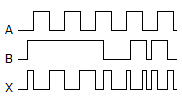# Online Digital Electronics Test - Digital Electronics Test 1Loading Test...

Instruction:

• This is a FREE online test. DO NOT pay money to anyone to attend this test.
• Total number of questions : 20.
• Time alloted : 30 minutes.
• Each question carry 1 mark, no negative marks.
• DO NOT refresh the page.
• All the best :-).

1.

What is the resultant binary of the decimal problem 49 + 01 = ?

A.
 01010101B.
 00110101C.
 00110010D.
 001100012.

Binary 10111111 is ________ in hexadecimal.

A.
 BF16B.
 FB16C.
 27716D.
 101111113.

An inverter output is the complement of its input.

A.
 TrueB.
 False4.

The following waveform pattern is for a(n) ________.A.
 2-input AND gateB.
 2-input OR gateC.
 Exclusive-OR gateD.
 None of the above5.

How many 3-line-to-8-line decoders are required for a 1-of-32 decoder?

A.
 1B.
 2C.
 4D.
 86.

The binary numbers A = 1100 and B = 1001 are applied to the inputs of a comparator. What are the output levels?

A.
 A > B = 1, A < B = 0, A < B = 1B.
 A > B = 0, A < B = 1, A = B = 0C.
 A > B = 1, A < B = 0, A = B = 0D.
 A > B = 0, A < B = 1, A = B = 17.

A logic probe is placed on the output of a gate and the display indicator is dim. A pulser is used on each of the input terminals, but the output indication does not change. What is wrong?

A.
 The output of the gate appears to be open.B.
 The dim indication on the logic probe indicates that the supply voltage is probably low.C.
 The dim indication is a result of a bad ground connection on the logic probe.D.
 The gate may be a tristate device.8.

Most people would prefer to use ________ over HDL.

A.
 graphic descriptionsB.
 functionsC.
 VHDLD.
 AHDL9.

When the output of the NOR gate S-R flip-flop is in the HOLD state (no change), the inputs are ________.

A.
 S = 1, R = 1B.
 S = 1, R = 0C.
 S = 0, R = 1D.
 S = 0, R = 010.

Erasing or programming a flash memory device is a one-step operation.

A.
 TrueB.
 False11.

DRAM uses a cross-transistor configuration.

A.
 TrueB.
 False12.

Which is a mode of operation of the GAL16V8?

A.
 Simple modeB.
 Complex modeC.
 Registered modeD.
 All of the above13.

Four subcategories of ASIC devices are available to create digital systems. These are PLDs, gate arrays, standard cells, and ________.

A.
 HCPLDsB.
 full customC.
 GALD.
 FPLDs14.

A NAND gate consists of an AND gate and an OR gate connected in series with each other.

A.
 TrueB.
 False15.

Solve this binary problem: 01000110 ÷ 00001010 =

A.
 0111B.
 10011C.
 1001D.
 001116.

Which is not a step used to define the scope of an HDL project?

A.
 Are the inputs and outputs active HIGH or active LOW?B.
 A clear vision of how to make each block workC.
 What are the speed requirements?D.
 How many bits of data are needed?17.

When coming up with a strategy for dividing the overall project into manageable-size pieces one must ________.

A.
 name each input and outputB.
 fully understand how the device should operateC.
 define successful completion of the projectD.
 know the nature of all the signals that interconnect all the pieces18.

In a real project, the first step of definition often involves some ________ on the part of the project manager.

A.
 timeB.
 skillC.
 researchD.
 management19.

The stepper motor HDL will ignore its counter inputs and pass control inputs directly to the output when set in mode ________.

A.
 1B.
 2C.
 3D.
 420.

The output of a basic 4-bit input digital-to-analog converter would be capable of outputting:

A.
 16 different values of voltage or current that are not proportional to the input binary numberB.
 16 different values of voltage or current that are proportional to the input binary numberC.
 32 different values of voltage or current that are not proportional to the input binary numberD.
 32 different values of voltage or current that are proportional to the input binary number